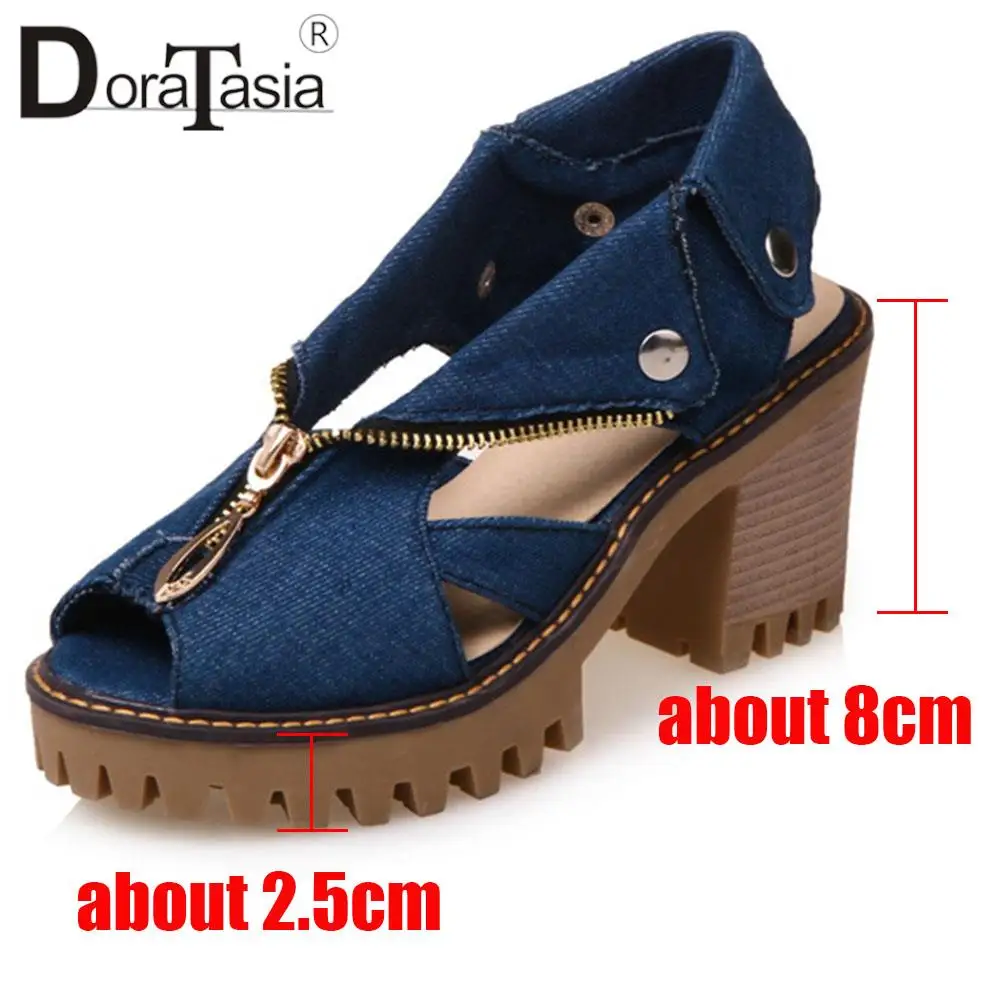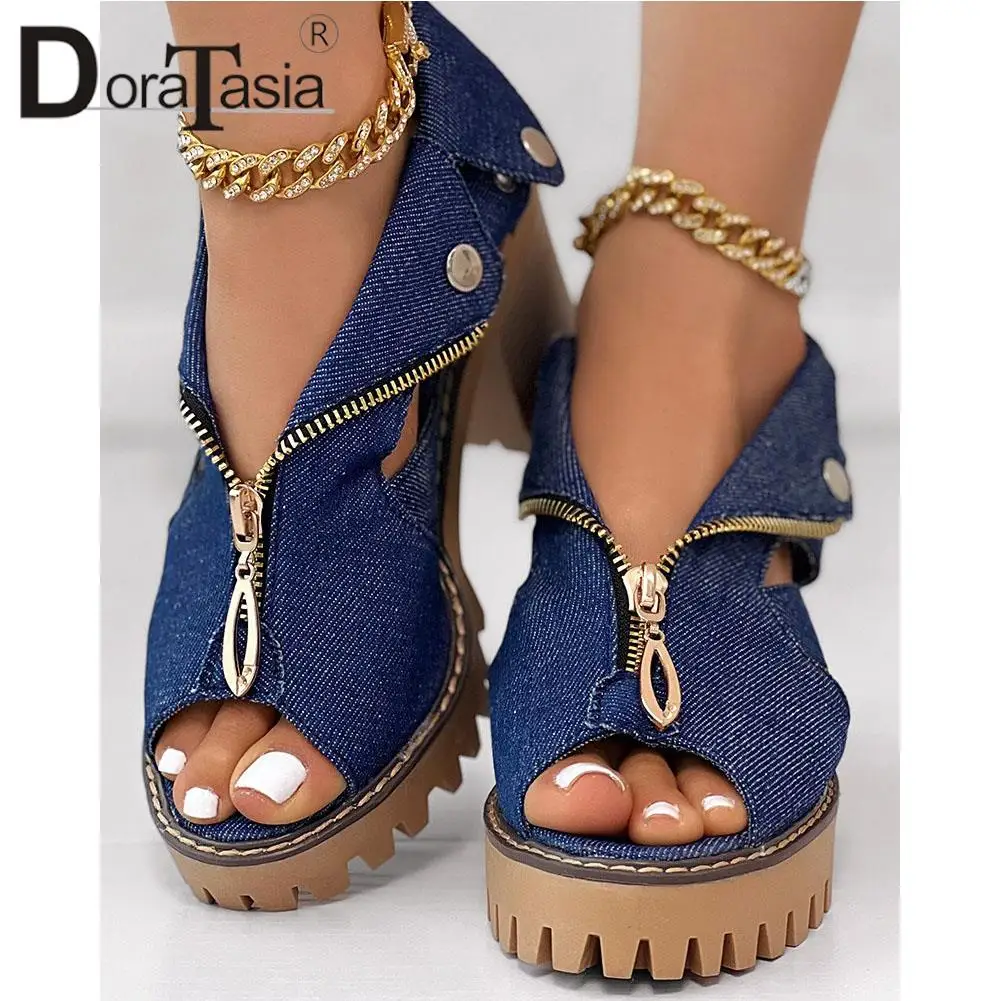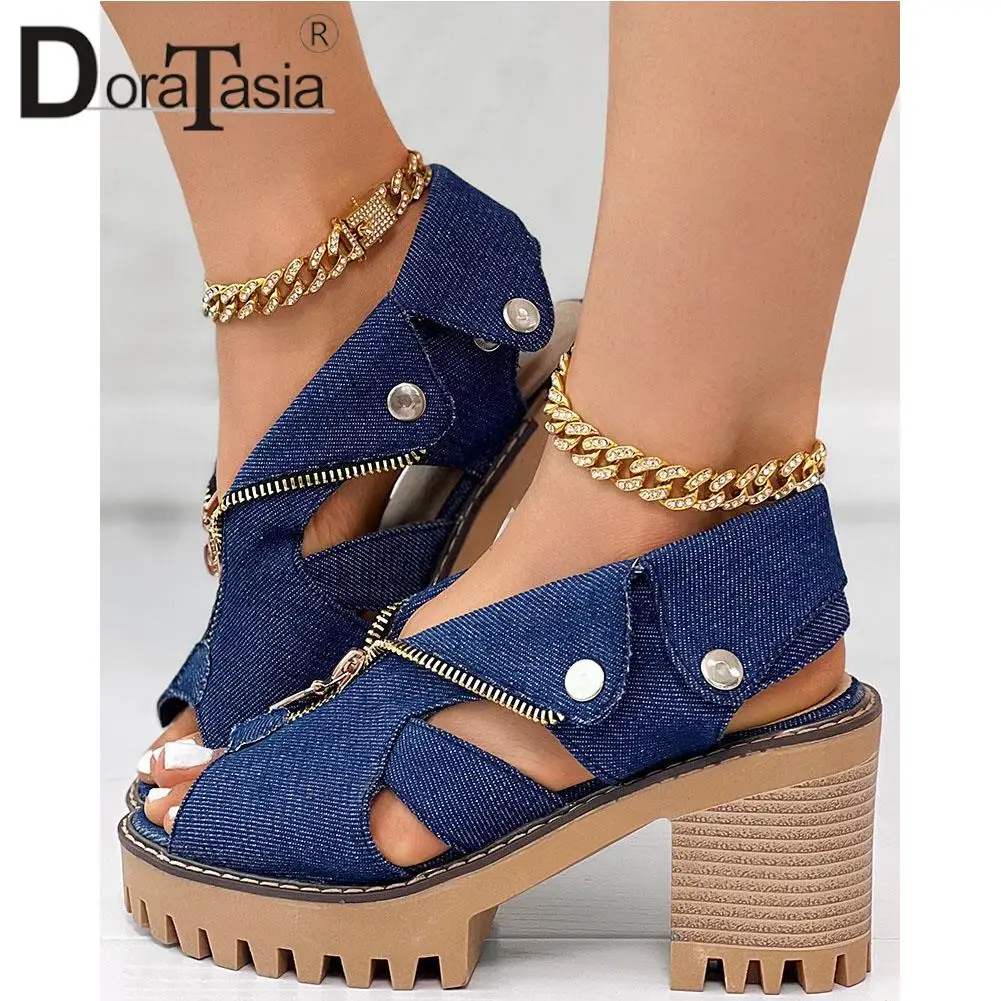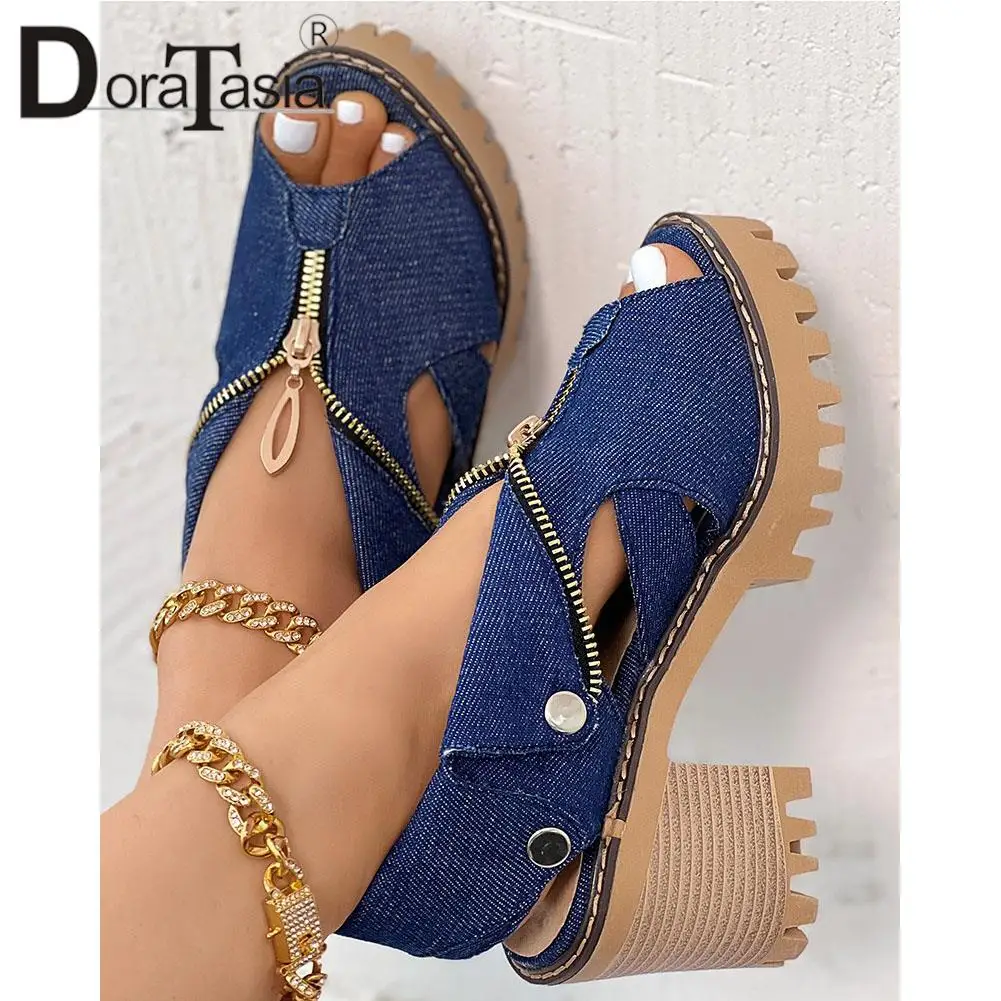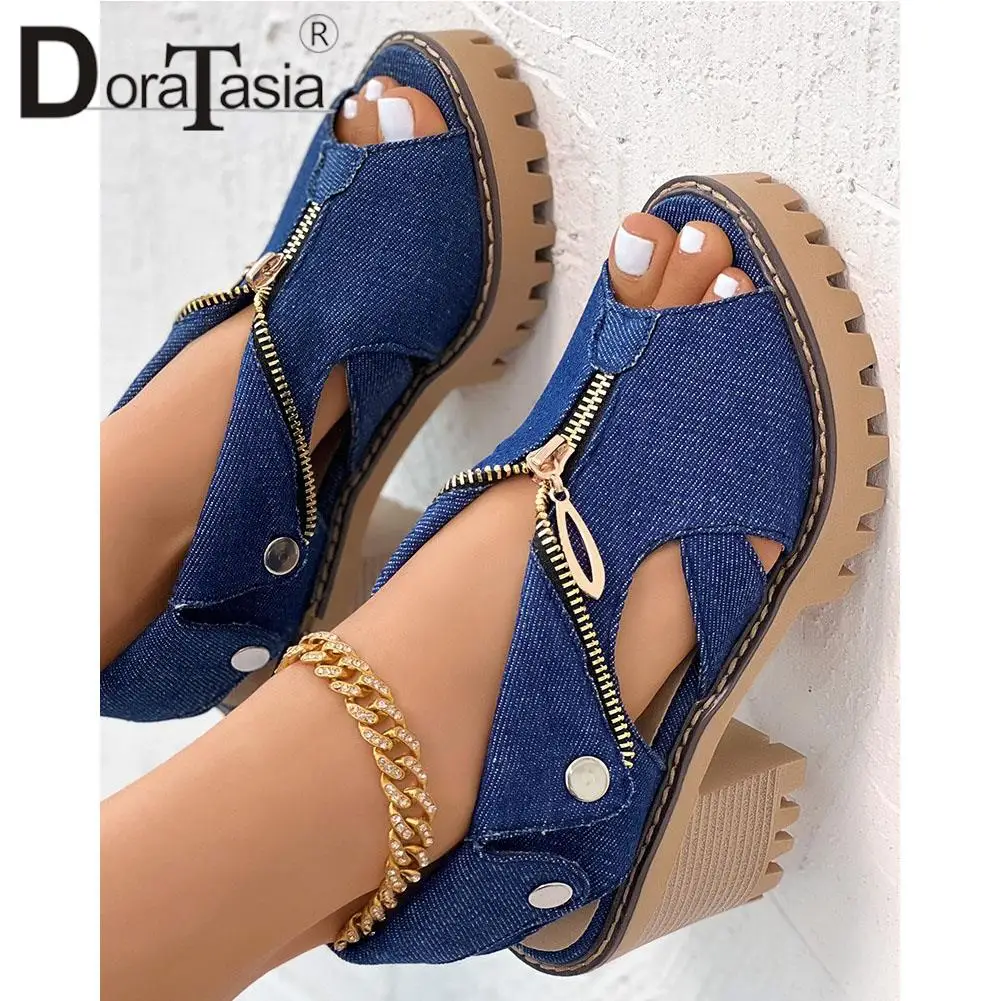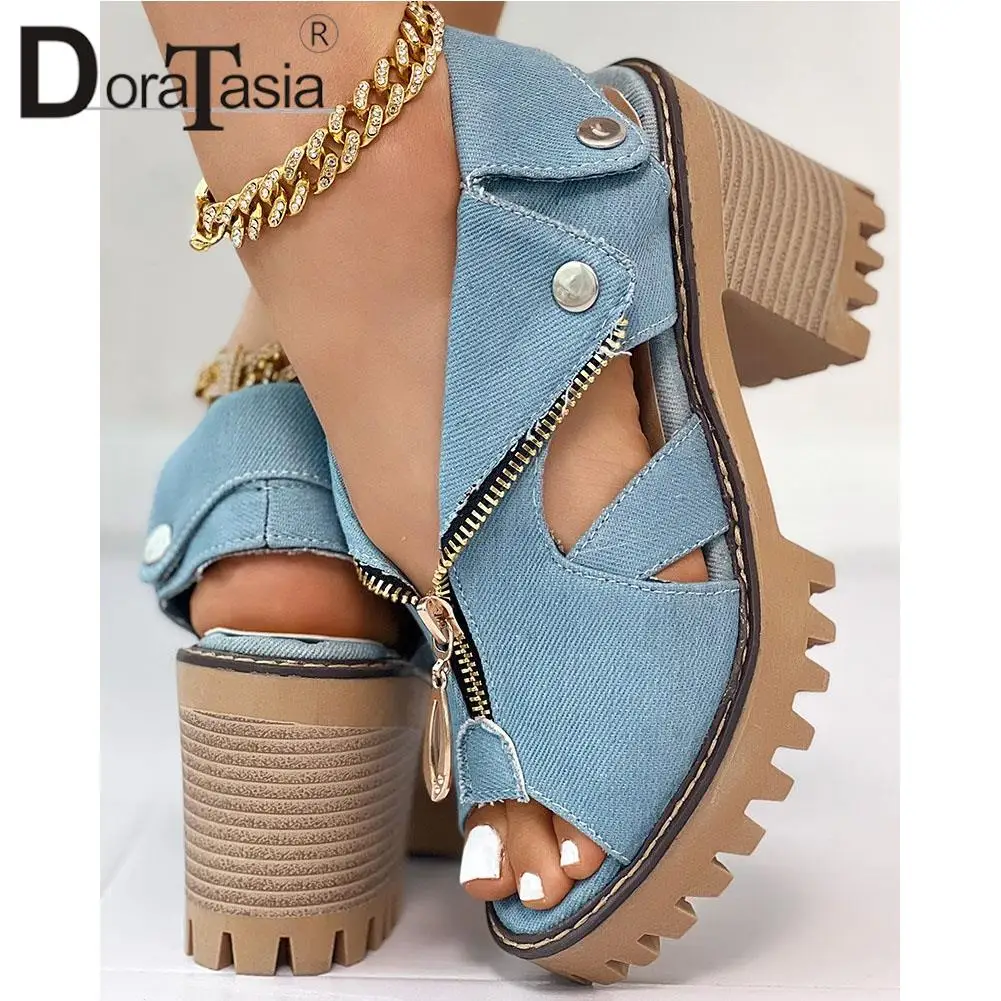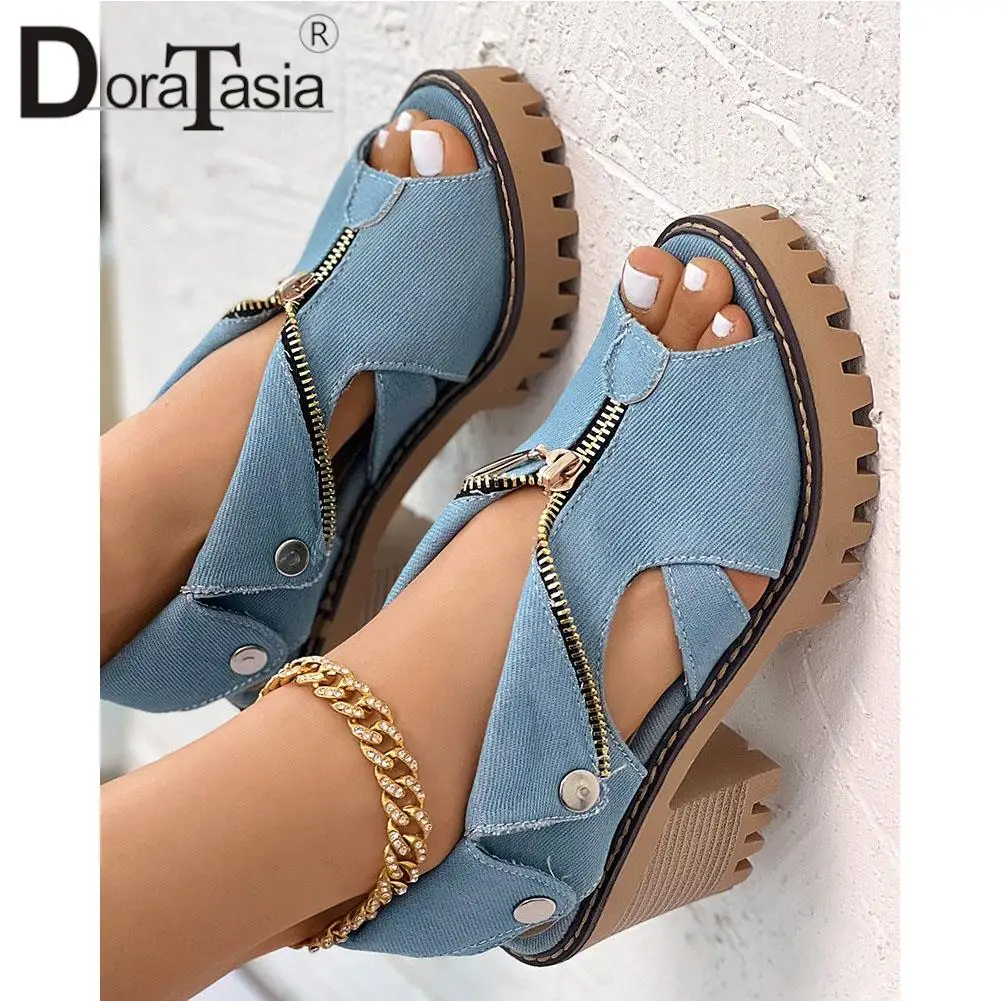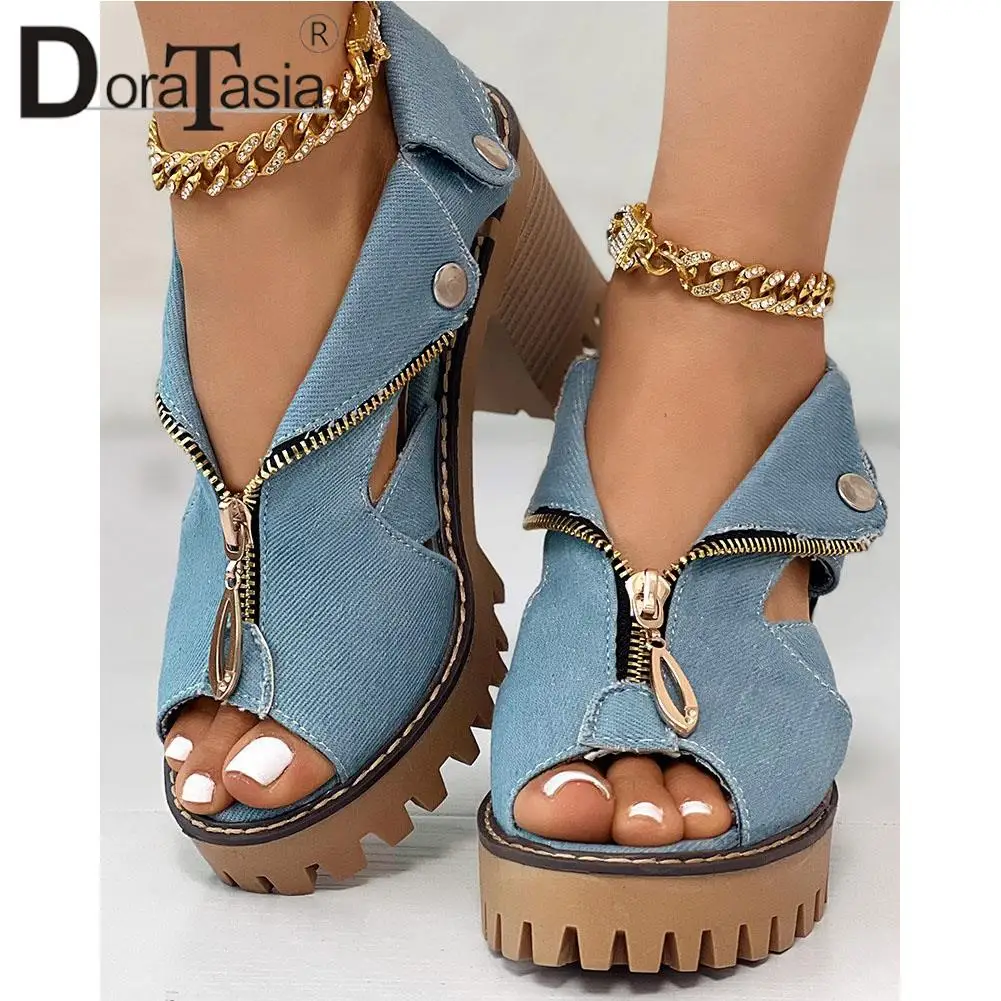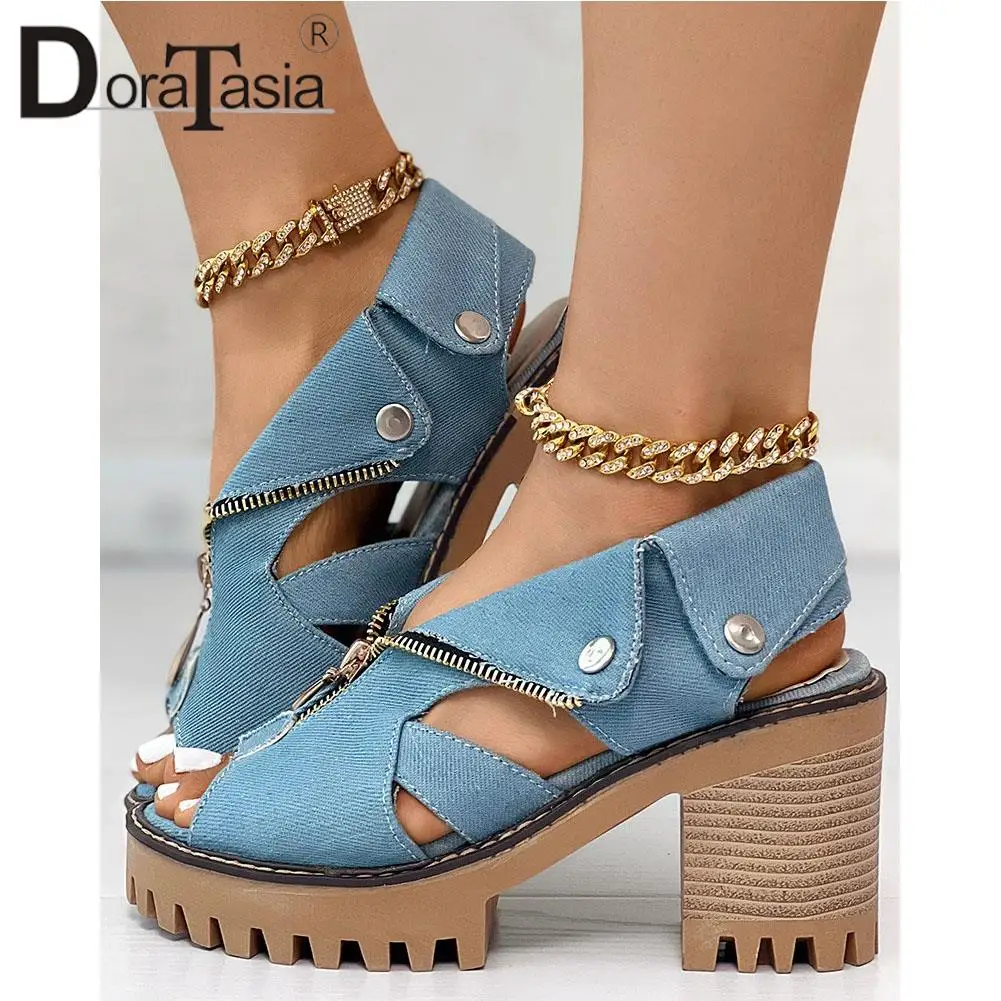# Martha - Denim Gladiator Sandals

Regular price \$134.99 \$92.99 Sale

US Size 5=CN35=225mm (bare feet length)=EUR35=UK2.5=Feet Length(8.86")
US Size 6=CN36=230mm (bare feet length)=EUR36=UK3.5=Feet Length(9.06")
US Size 6.5=CN37=235mm (bare feet length)=EUR36.5=UK4=Feet Length(9.25")
US Size 7.5=CN38=240mm (bare feet length)=EUR37=UK5=Feet Length(9.45")
US Size 8.5=CN39=245mm (bare feet length)=EUR38=UK6=Feet Length(9.65")
US Size 9=CN40=250mm (bare feet length)=EUR39=UK6.5=Feet Length(9.84")
US Size 9.5=CN41=255mm (bare feet length)=EUR40=UK7=Feet Length(10.04")
US Size 10=CN42=260mm (bare feet length)=EUR40.5=UK8=Feet Length(10.24")
US Size 10.5=CN43=265mm (bare feet length)=EUR41=UK8.5=Feet Length(10.43")